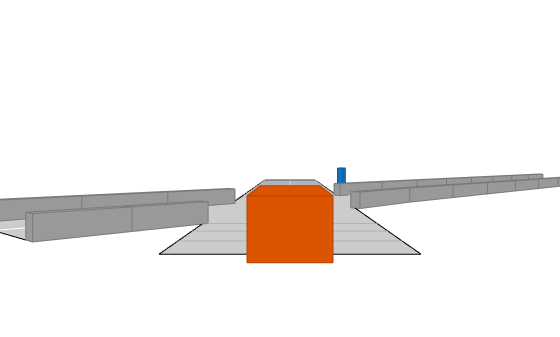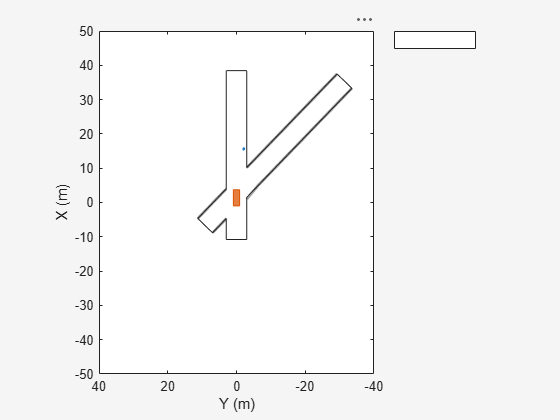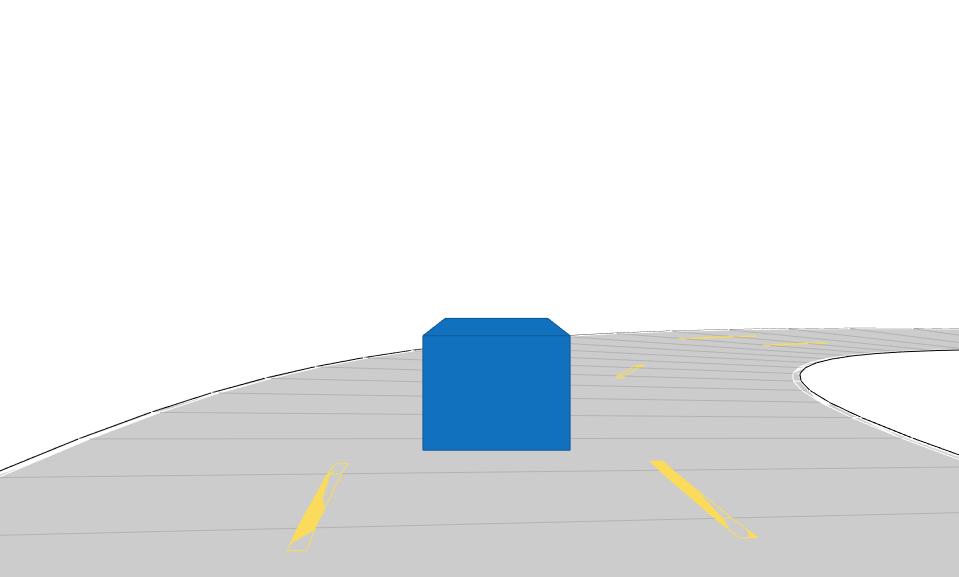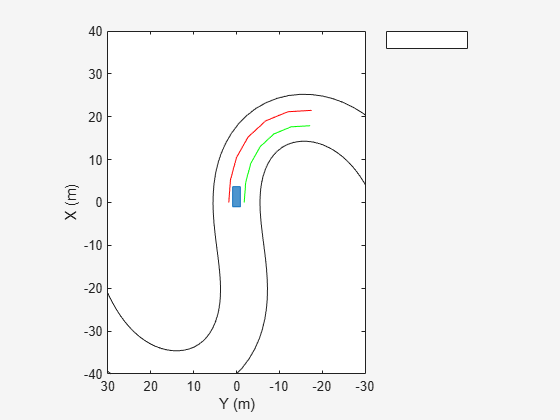Documentation

# trajectory

Create actor or vehicle trajectory in driving scenario

## Syntax

``trajectory(ac,waypoints,speed)``

## Description

example

````trajectory(ac,waypoints,speed)` creates a trajectory for an actor or vehicle, `ac`, from a set of waypoints. The actor follows the trajectory at the specified speed.```

## Examples

collapse all

Create a driving scenario and add a curved two-lane road to it.

```scenario = drivingScenario('SampleTime',0.05); roadcenters = [5 0; 30 10; 35 25]; lspec = lanespec(2); road(scenario,roadcenters,'Lanes',lspec); ```

Add a vehicle to the scenario. Set a trajectory in which the vehicle drives around the curve at varying speeds.

```v = vehicle(scenario); waypoints = [6 2; 18 4; 25 7; 28 10; 31 15; 33 22]; speeds = [30 10 5 5 10 30]; trajectory(v,waypoints,speeds) ```

Plot the scenario and run the simulation. Observe how the vehicle slows down as it drives along the curve.

```plot(scenario,'Waypoints','on','RoadCenters','on') while advance(scenario) pause(0.1) end ```Create a driving scenario and show how target outlines change as the simulation advances.

Create a driving scenario consisting of two intersecting straight roads. The first road segment is 45 meters long. The second straight road is 32 meters long and intersects the first road. A car traveling at 12.0 meters per second along the first road approaches a running pedestrian crossing the intersection at 2.0 meters per second.

```scenario = drivingScenario('SampleTime',0.1,'StopTime',1); road(scenario,[-10 0 0; 45 -20 0]); road(scenario,[-10 -10 0; 35 10 0]); ped = actor(scenario,'Length',0.4,'Width',0.6,'Height',1.7); car = vehicle(scenario); pedspeed = 2.0; carspeed = 12.0; trajectory(ped,[15 -3 0; 15 3 0],pedspeed); trajectory(car,[-10 -10 0; 35 10 0],carspeed);```

Create an ego-centric chase plot for the vehicle.

`chasePlot(car,'Centerline','on')`Create an empty bird's-eye plot and add an outline plotter and lane boundary plotter. Then, run the simulation. At each simulation step:

• Update the chase plot to display the road boundaries and target outlines.

• Update the bird's-eye plot to display the updated road boundaries and target outlines. The plot perspective is always with respect to the ego vehicle.

```bepPlot = birdsEyePlot('XLim',[-50 50],'YLim',[-40 40]); outlineplotter = outlinePlotter(bepPlot); laneplotter = laneBoundaryPlotter(bepPlot); legend('off') while advance(scenario) rb = roadBoundaries(car); [position,yaw,length,width,originOffset,color] = targetOutlines(car); plotLaneBoundary(laneplotter,rb) plotOutline(outlineplotter,position,yaw,length,width, ... 'OriginOffset',originOffset,'Color',color) pause(0.01) end```Simulate a driving scenario with one car traveling on an S-curve. Create and plot the lane boundaries.

Create the driving scenario with one road having an S-curve.

```scenario = drivingScenario('StopTime',3); roadcenters = [-35 20 0; -20 -20 0; 0 0 0; 20 20 0; 35 -20 0]; ```

Create the lanes and add them to the road.

```lm = [laneMarking('Solid','Color','w'); ... laneMarking('Dashed','Color','y'); ... laneMarking('Dashed','Color','y'); ... laneMarking('Solid','Color','w')]; ls = lanespec(3,'Marking',lm); road(scenario,roadcenters,'Lanes',ls); ```

Add an ego vehicle and specify its trajectory from its speed and waypoints. The car travels at 30 meters per second.

```car = vehicle(scenario, ... 'ClassID',1, ... 'Position',[-35 20 0]); waypoints = [-35 20 0; -20 -20 0; 0 0 0; 20 20 0; 35 -20 0]; speed = 30; trajectory(car,waypoints,speed); ```

Plot the scenario and corresponding chase plot.

```plot(scenario) ``````chasePlot(car) ```Run the simulation loop.

1. Initialize a bird's-eye plot and create an outline plotter, left-lane and right-lane boundary plotters, and a road boundary plotter.

2. Obtain the road boundaries and rectangular outlines.

3. Obtain the lane boundaries to the left and right of the vehicle.

4. Advance the simulation and update the plotters.

```bep = birdsEyePlot('XLim',[-40 40],'YLim',[-30 30]); olPlotter = outlinePlotter(bep); lblPlotter = laneBoundaryPlotter(bep,'Color','r','LineStyle','-'); lbrPlotter = laneBoundaryPlotter(bep,'Color','g','LineStyle','-'); rbsEdgePlotter = laneBoundaryPlotter(bep); legend('off'); while advance(scenario) rbs = roadBoundaries(car); [position,yaw,length,width,originOffset,color] = targetOutlines(car); lb = laneBoundaries(car,'XDistance',0:5:30,'LocationType','Center', ... 'AllBoundaries',false); plotLaneBoundary(rbsEdgePlotter,rbs) plotLaneBoundary(lblPlotter,{lb(1).Coordinates}) plotLaneBoundary(lbrPlotter,{lb(2).Coordinates}) plotOutline(olPlotter,position,yaw,length,width, ... 'OriginOffset',originOffset,'Color',color) end ```## Input Arguments

collapse all

Actor belonging to a `drivingScenario` object, specified as an `Actor` or `Vehicle` object. To create these objects, use the `actor` and `vehicle` functions, respectively.

Trajectory waypoints, specified as a real-valued N-by-2 or N-by-3 matrix, where N is the number of waypoints.

• If `waypoints` is an N-by-2 matrix, then each matrix row represents the (x, y) coordinates of a waypoint. The z-coordinate of each waypoint is zero.

• If `waypoints` is an N-by-3 matrix, then each matrix row represents the (x, y, z) coordinates of a waypoint.

Waypoints are in the world coordinate system. Units are in meters.

Example: `[1 0 0; 2 7 7; 3 8 8]`

Data Types: `double`

Actor speed at each waypoint in `waypoints`, specified as a positive real scalar or N-element vector of nonnegative values. N is the number of waypoints.

• When `speed` is a scalar, the speed is constant throughout the actor motion.

• When `speed` is a vector, the vector values specify the speed at each waypoint.

Speeds are interpolated between waypoints. `speed` can be zero at any waypoint but cannot be zero at two consecutive waypoints. Units are in meters per second.

Example: `[10,8,9]`

## Algorithms

The `trajectory` function creates a trajectory for an actor to follow in a scenario. A trajectory consists of the path followed by an object and its speed along the path. You specify the path using N two-dimensional or three-dimensional waypoints. Each of the N – 1 segments between waypoints defines a curve whose curvature varies linearly with distance along the segment. The function fits a piecewise clothoid curve to the (x, y) coordinates of the waypoints by matching the curvature on both sides of the waypoint. For a nonclosed curve, the curvature at the first and last waypoint is zero. If the first and last waypoints coincide, then the curvatures before and after the endpoints are matched. The z-coordinates of the trajectory are interpolated using a shape-preserving piecewise cubic curve.

You can specify speed as a scalar or a vector. When speed is a scalar, the actor follows the trajectory with constant speed. When speed is an N-element vector, speed is linearly interpolated between waypoints. Setting the speed to zero at two consecutive waypoints creates a stationary actor.